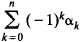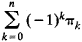# Euler Characteristic

(redirected from Euler's polyhedron theorem)

## Euler Characteristic

The Euler characteristic of a polyhedron is the number α0 — α1 + α2, where α0 is the number of vertices, α1 is the number of edges, and α2 is the number of faces. According to Euler’s theorem, if the polyhedron is convex or is homeomorphic to a convex polyhedron, then its Euler characteristic is 2. This fact was known to R. Descartes; L. Euler published a proof of the theorem in 1758.

The Euler characteristic of an arbitrary simplicial complex is the numberwhere n is the dimension of the complex, α0 is the number of vertices, and α1 is the number of edges. In general, k is the number of k-dimensional simplexes belonging to the complex. According to the Euler-Poincaré formula, the Euler characteristic is equal towhere πk is the k-dimensional Betti number of the complex. The topological invariance of the Euler characteristic follows from this fact.

In view of the topological invariance of the Euler characteristic, we speak of the Euler characteristic of a surface and of a polytope, by which we mean the Euler characteristic of any triangulation of the surface or polytope.

### REFERENCES

Aleksandrov, P. S. Kombinatornaia topologiia. Moscow-Leningrad, 1947.
Pontriagin, L. S. Osnovy kombinatornoi topologii, 2nd ed. Moscow, 1976.
Site: Follow: Share:
Open / Close